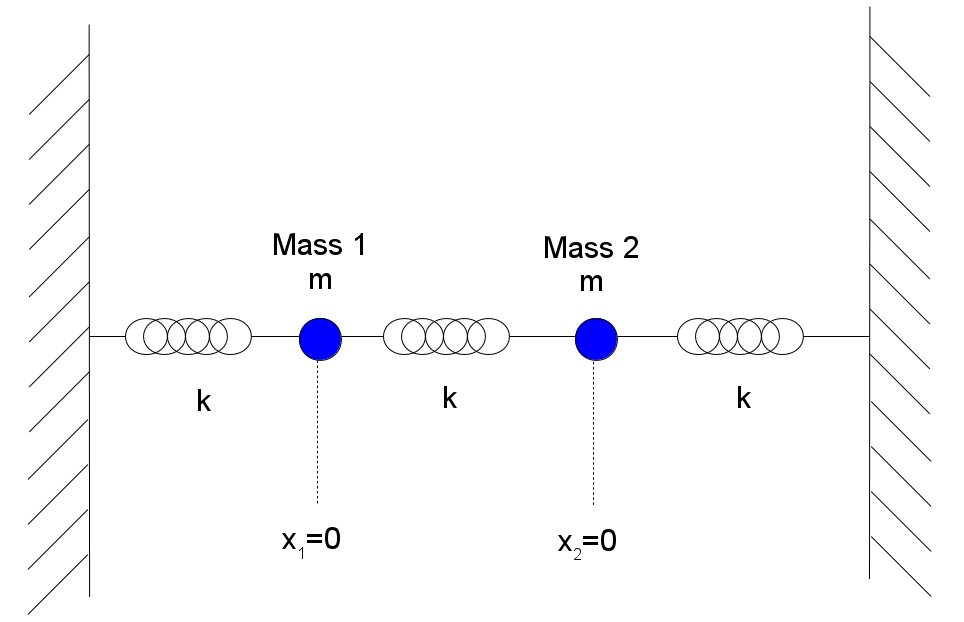# How to calculate the potential energy of coupled oscillators?The equations of motion that describe the above situation is given by:

$$m \ddot{x_1} = -2kx_1 + kx_2$$

$$m \ddot{x_2} = -2kx_2 + kx_1$$

Now I want to work out the potential energy of this system. How would I use the equation:

$$V(x) = - \int F(x) \hspace{.1cm} dx$$

to calculate the potential energy?

My attempt is to first calculate the integral for each coupled equation wrt each position:

$$- \int (- 2kx_1 + + kx_2) \hspace{.1cm} dx_1= kx^{2}_1 - k x_1 x_2 + c$$ $$- \int (- 2kx_2 + + kx_1) \hspace{.1cm} dx_2= kx^{2}_2 - k x_2 x_1 + d.$$

Now adding them together to get the total potential energy gives:

$$V(x_1, x_2) = kx^{2}_1 + kx^{2}_2 + - 2 k x_1 x_2 + f.$$

The actual solution is given by:

$$V(x_1, x_2) = kx^{2}_1 + kx^{2}_2 + - k x_1 x_2$$

Now I have inkling as to why my answer is wrong. When adding the potentials together to calculate the total potential I have double added the potential associated with the middle spring. However, mathematically how do I fix this issue to get the correct solution?

• Remember that a spring has energy $\frac 12 k(x_{1}-x_0 -a)^2$ where $a$ is it's natural length. – mike stone Oct 1 at 13:54
• – Frobenius Oct 1 at 18:26

As @mikestone suggested in the comments, the easiest way to solve this problem is by summing the potential energies of all the springs.

However, I would like to comment on the approach described in the question: the equations of motion can be written as $$m\ddot{x}_1 = -\frac{\partial V(x_1, x_2)}{\partial x_1} = -2kx_1 + kx_2,\\ m\ddot{x}_2 = -\frac{\partial V(x_1, x_2)}{\partial x_2} = -2kx_2 + kx_1,$$ where $$V(x_1, x_2)$$ is the potential energy of the two oscillators. We thus have two partial differential equations (pde) for this potential energy. Integrating the first one in respect to $$x_1$$ we obtain: $$V(x_1, x_2) = kx_1^2 - kx_2x_1 + C(x_2),$$ i.e. we obtain $$V(x_1, x_2)$$ up to an unknown constant, $$C(x_2)$$, which may be dependent on $$x_2$$ (since the differential equation is in respect to $$x_1$$). Substituting this into the second equation we obtain: $$\frac{\partial V(x_1, x_2)}{\partial x_2} = -kx_1 +\frac{dC(x_2)}{dx_2} = 2kx_2 - kx_1,$$ that is $$\frac{dC(x_2)}{dx_2} = 2kx_2 \Rightarrow C(x_2) = kx_2^2$$ (up to a constant independent on either $$x_1$$ or $$x_2$$), and we obtain $$V(x_1, x_2) = kx_1^2 + kx_2^2 -kx_1x_2.$$

• Thank you that makes perfect sense. – Nice1m80 Oct 1 at 14:21

Question Can be Solved without Integration. Potential Energy is stored in the springs only.Let The Rightmost spring is stretched by amount $$x_2$$, And the Leftmost spring is stretched by amount $$x_1$$ then the Middle spring will be stretched by amount $$(x_2 - x_1)$$.($$As\ shown\ in \ figure$$) $$(2a +x_2)-(a+ x_1)= a+ x_2 - x_1 \qquad (Proof)$$

where a is the length of spring.

The Potential energy of the spring is given by $$V(x) = \frac{1}{2}kx^{2}$$

Potential energy of leftmost spring is $$V(x_1) = \frac{1}{2}kx^{2}_1 \qquad ...(1)$$

Potential energy of rightmost spring is $$V(x_2) = \frac{1}{2}kx^{2}_2 \qquad ...(2)$$

Potential energy of Middle spring is $$V(x_3) = \frac{1}{2}k(x_1-x_2)^{2}\qquad ...(3)$$

$$V(x)=V(x_1)+V(x_2)+V(x_3)$$

$$V(x) = kx^{2}_1 + kx^{2}_2 - kx_1x_2$$

• For the middle spring $(x_1-x_2)^2$ in eq. 3 – Vadim Oct 1 at 16:09

Here's a slightly different take on someone else's answer that does not assume the spring constants are necessarily the same. It also uses the inspection method, based on the idea that the potential energy of a spring is $$\frac{1}{2}\kappa \times \hbox{stretch}^2$$ (or compression).

For your first spring on the left, the stretch would come from the displacement $$x_1$$ so $$V_1=\frac{1}{2}\kappa_1 x_1^2$$. For the spring in the middle the stretch is $$\vert x_1-x_2\vert$$ so you'd get $$V_2=\frac{1}{2}\kappa_2(x_1-x_2)^2$$. For the spring on the right $$V_3=\frac{1}{2}\kappa_3 x_2^2$$.

Then it's just a matter of finding the $$\kappa_i$$'s using the equations of motion: \begin{align} m\ddot{x_1}&=-\kappa_1 x_1 -\kappa_2 (x_1-x_2)=-(\kappa_1+\kappa_2)x_2+\kappa_2x_2\, ,\\ m\ddot{x_2}&=\kappa_2(x_1-x_2)-\kappa_3 x_2=-(\kappa_2+\kappa_3)x_2+\kappa_2 x_1 \end{align}

Direct comparison with your EOMs yields $$\kappa_2=\kappa_1=\kappa_3=k$$ so the net potential energy is $$V=V_1+V_2+V_3=\frac{1}{2}k\left(x_1^2+x_2^2+(x_1-x_2)^2\right)\, .$$